# NCERT Solutions for Class 12 Chemistry Chapter 4 Chemical Kinetics

Here we provide NCERT Solutions for Class 12 Chemistry Chapter 4 Chemical Kinetics for English medium students, Which will very helpful for every student in their exams. Students can download the latest NCERT Solutions for Class 12 Chemistry Chapter 4 Chemical Kinetics pdf, free NCERT solutions for Class 12 Chemistry Chapter 4 Chemical Kinetics book pdf download. Now you will get step by step solution to each question.

## NCERT Solutions for Class 12 Chemistry Chapter 4 Chemical Kinetics

Class 12 Chemistry chapter 4 Chemical Kinetics is an important chapter and is often asked in the examination. Chemical kinetics helps to understand the chemical reactions. This chapter explains all about the rate of reaction and the factors determining the rate of reaction.

NCERT IN-TEXT QUESTIONS

Question 1.
For a reaction R → P, the concentration of a reactant changes from 0·03 M to 0·02 M in 25 minutes. Calculate the average rate of the reaction using the units of seconds.
For a reaction, R → P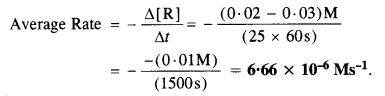Question 2.
In a reaction, 2A → Products, the concentration of A decreases from 0·5 mol L-1 to 0·4 mol L-1 in 10 minute. Calculate the rate during this interval.
For the reaction: 2A → ProductsQuestion 4.
Fora reaction,A+B —> Product; the rate law is given by, r =k [ A]1/2 [B]2. What is the order of the reaction?
Order of reaction. = 1/2+ 2 = 21/2 or 2.5

Question 4.
The conversion of the molecules X to Y follows second order kinetics. If concentration of X is increased to three times, how will it affect the rate of formation of Y ?
For the reaction X → Y
Reaction rate (r) = k[X]2
If the concentration be increased to three times, then
Reaction rate (r’) = k [3X]2
(frac { { r }^{ ‘ } }{ r } =frac { kleft[ 3X right] ^{ 2 } }{ kleft[ X right] ^{ 2 } } =9)

Question 5.
A first order reaction has rate constant of 1·15 x 10-3 s-1. How long will 5 g of this reactant take to reduce to 3g?
For the first order reaction,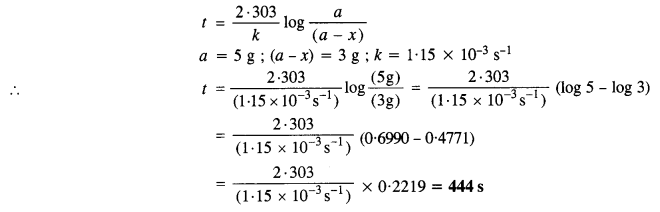Question 6.
Time required to decompose SO2Cl2 to half of its initial concentration is 60 minutes. If the decomposition is a first order reaction, calculate the rate constant of the reaction.
For the first order reaction ;
Rate constant (k) = (frac { 0.693 }{ { t }_{ 1/2 } } =frac { 0.693 }{ left( 60min right) } )
= (frac { 0.693 }{ left( 60times 60s right) } =1.925times { 10 }^{ -4 }{ s }^{ -1 })

Question 7.
What will be the effect of temperature on rate constant?
With the rise in temperature by 10°, the rate constant of a reaction is nearly doubled. The dependence of rate constant on temperature is given the Arrhenius equation, k = A e-Ea/RT where A is the Arrhenius constant and Ea is activation energy of the reaction.

Question 8.
In general, it is observed that the rate of a chemical reaction doubles with every 10° rise in temperature. If the generalisation holds for a reaction in the temperature range 295 K to 305 K, what would be the value of activation energy for the reaction? (C.B.S.E. Delhi2005 Supp., Pb. Board2007)
According to Arrhenius equation,Question 9.
The activation energy for the reaction, 2HI(g) → H2(g) + I2(g) is 208·5 kJ mol-1. Calculate fraction of molecules of reactants having energy equal to or greater than activation energy.
The fraction of the molecules (x) having energy equal to or more than activation energy may be calculated as follows :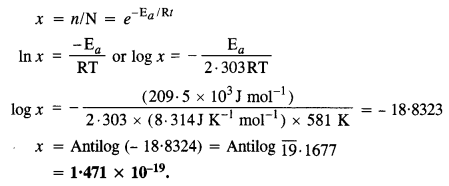NCERT Exercise

Question 1.
From the rate expression for the following reactions determine the order of reaction and the dimensions of the rate constant.(a) Order of reaction = 2
Dimension of rate constant (k) = [mol L-1]1-ns-1 = [mol L-1](1-2)s-1 = mol-1 L s-1

(b) Order of reaction = 1 + 1=2
Dimension of rate constant (k) = [mol L-1]1-ns-1 = [mol L-1](1-2)s-1 = mol-1 L s-1

(c) Order of reaction = 3/2
Dimension of rate constant (k) = [mol L-1]1-n s-1 = [mol L-1]1-3/2s-1 = mol-1/2L1/2s-1

(d) Order of reaction = 1 + 1/2 = 3/2
Dimension of rate constant (k) = [mol L-1]1-n s-1 = [mol L-1]1-3/2s-1 = mol-1/2L1/2s-1

(e) Order of reaction = 1
Dimension of rate constant (k) = [mol L-1]1-n s-1 = [mol L-1](1-1)s-1 = s-1

Question 2.
For the reaction, 2A + B ————> A2 B, the rate = k [AJ[B]2 with k = 2.0 x 10-6 mol-2 L2 s-1. Calculate the initial rate of the reaction when [A] = 0.1 mol L-1, [B] = 0.2 mol L-1. Calculate the rate of reaction after [A] is reduced to 0.06 mol L-1.
Initial rate of reaction = k [A] [B]2
= (20 x 10-6 mol-2 s-1) (0.1 mol L-1) (0.2 mol L-1)2 = 8 x 10-9molL-1 s-1.
When [A] is reduced from 010 mol L-1 to 0.06 molL-1, i.e., 0.04 mol L-1 of A has reacted, the concentration of B reacted, is = 1/2 x 0.04 mol L-1 = 0.02 mol L-1
Concentration of B, remained after reaction with A = 0.2 – 0-02=0.18 mol L-1
Now, rate=(20 x 10-6 mol-2 L2 s-1) (0.06 mol L-1) (0.18 molL-1)2
= 3-89 x 10-9mol L-1 s-1

Question 3.
The rate of decomposition of NH3 on platinum surface is zero order. What are rate of production of N2 and H2 if k = 2·5 x 10-4 Ms-1 ? (C.B.S.E. Delhi 2008)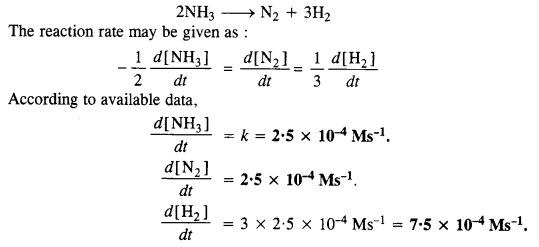Question 4.
The decomposition of dimethyl ether leads to the formation of CH4, H2 and CO and the reaction rate is given by the expression :
rate = k [CH3OCH3]3/2
The rate of reaction is followed by increase in pressure in a close vessel and the rate can also be expressed in terms of partial pressure of dimethyl ether :
rate = k [pCH3OCH3]3/2
If the pressure is measured in bar and time in minutes, then what are the units of rate and rate constant ?Question 5.
Mention the factors that affect the rate of a chemical reaction.
Factors affecting the rate 6f chemical reaction are following:
(i) Natpre of reactants
(ii) Concentration of reactants
(iii) Surface area of reactants
(iv) Effect of temperature
(v) Presence of catalyst
(vi) Presence of light

Question 6.
A reaction is second order with respect to a reactant. How is the rate of reaction affected if the concentration of the reactant is
(i) doubled
(ii) reduced to 1/2 ? (C.B.S.E. Outside Delhi 2008, 2009)
Let the reaction be ; A → Products
Reaction rate (r) = k [A]2 (for second order reaction)
(i) When concentration is doubled, the rate of reaction may be expressed as :
Reaction rate (r’) = k [2A]2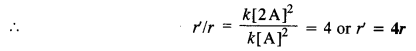reaction rate becomes four times.
(ii) When concentration is reduced to half, the rate of reaction may be expressed as :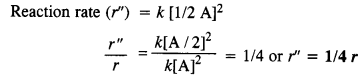reaction rate will be reduced to 1/4.

Question 7.
What is the effect of temperature on the rate constant of a reaction? How can this effect of temperature on rate constant be represented quantitatively?
The rate constant of a reaction increases with increase of temperature and becomes nearly double for every 10 °C rise of temperature. Quantitatively, this temperature effect on the rate constant is represented as, k = Ae-Ea/RT
where E is the activation energy of the reaction, and A represents the frequency factor.

Question 8.
In a pseudo first order hydrolysis of ester in water, the following results were obtained.(i) Calculate the average rate of reaction between the time interval 30 to 60 seconds.
(ii) Calculate the pseudo first order rate constant for the hydrolysis of ester.Question 9.
A reaction is first order in A and second order in B
(i) Write differential rate equation.
(ii) How is rate affected when the concentration of B is tripled ?
(iii) How is rate affected when the concentration of both A and B are doubled ? (C.B.S.E. Outside Delhi 2010, 2013)Question 10.
In a reaction between A and B, the initial rate of reaction was measured for different initial concentration of A and B as given ahead :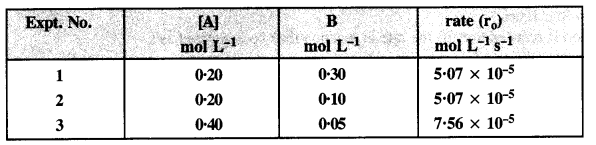What is the order of reaction with respect to A and B ?Question 11.
The following data were obtained at 300 K for the reaction 2A + B → C + D :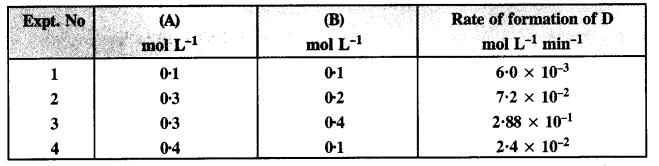Calculate the rate of formation of D when [A] = 0·5 mol L-1 and [B] = 0·2 mol L-1.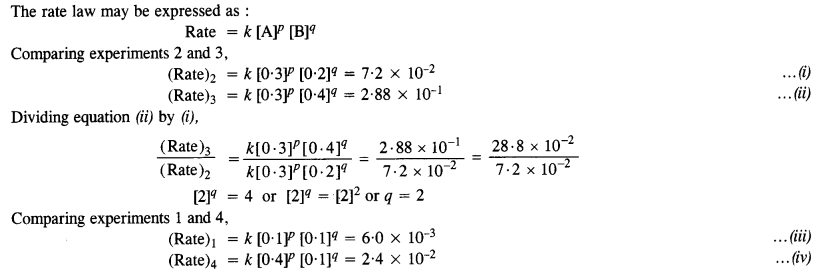Question 12.
The reaction between A and B is first order with respect to A and zero order with respect to B. Fill in the blanks in the following table :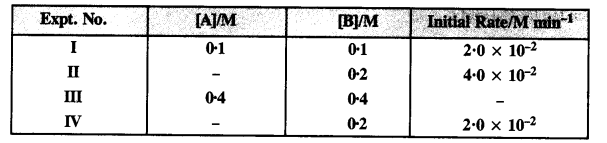The rate equation for the reaction is : r = k [A]1 [B]0
(i) Comparing experiments I and II,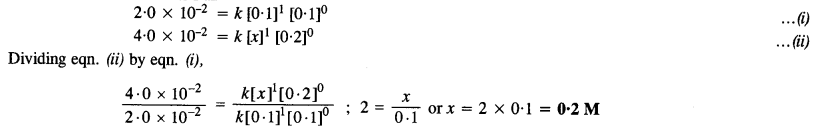Thus, concentration of A in experiment II is 0·2 M
(ii) Comparing experiments II and in.
When the concentration of A is made double, the reaction rate will also become twice.
∴ Rate of reaction in experiment III is 8·0 x 10-2
(iii) Comparing experiments I and IV.
Since the reaction rates are the same in both the experiments, the molar concentration of A in experiment IV must be the same as in experiment I i. e., it must be 0·1 M.

Question 13.
Calculate the half life of the first order reaction from their rate constants given as
(a) 200 s-1
(b) 2 min-1
(c) 4 year-1.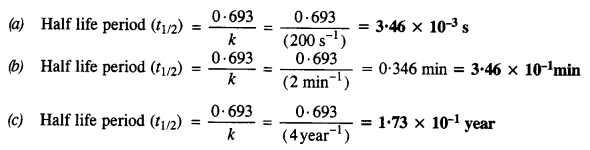Question 14.
The half life for radioactive decay of 14C is 5730 Y. An archaeological artifact contained wood had only 80% of the 14 C found in a living tree. Estimate age of the sample. (C.B.S.E. Delhi 2008)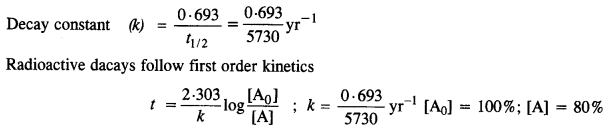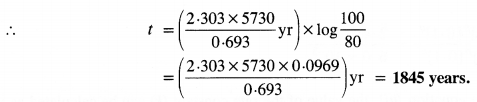Question 15.
The experimental data for decomposition of N2O2 [2N2O5 → 4NO2 + O2] in gas phase at 318 K are given below :(a) Plot [N2O5] against t
(b) Find the half-life period for the reaction
(c) Draw a graph between log [N2O5] and t
(d) What is rate law ?
(e) Calculate the rate constant
(f) Calculate the half-life period from k and compare it with (b).
The available data is :(b) Initial cone. of N2O5 = 1·63 x 10-2M. Half of initial cone. = 1/2 x (1·63 x 10-2 M) = 0·815 x 10-2 M Time corresponding to half of inital concentration (t/2) from the plot (a) = 1400 (s) approximately
(c) The graph of log [N2O5] Vs. time has been plotted.
(d) Since the graph between log [N2O5] and time is a straight line the reaction is of first order
The rate equation : rate (r) = k[N2O5]Question 16.
The rate constant for the first order reaction is 60 s-1. How much time will it take to reduce the initial concentration of the reactant to its 1/16th value ? (C.B.S.E. Delhi 2013)
For the first order reaction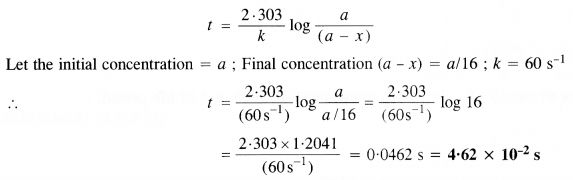Question 17.
During nuclear explosion, one of the products is 90Sr with half period of 28·1 Y. If 1 pg of 90Sr was absorbed in the bones of a newly born baby instead of calcium, how much of it will remain after 10 years and 60 years if it is not lost metabolicallv ?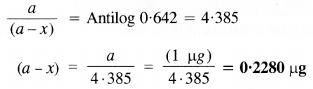Question 18.
For a first order reaction, show that time required for 99% completion is twice the time required for the completion of 90% of reaction.

Question 19.
A first order reaction takes 40 minutes for 30% decomposition. Calculate its half life period. (C. B. S. E. Outside Delhi 2013)

Question 20.
For the decomposition of azoisopropane to hexane and nitrogen at 543 K, the following data is obtained.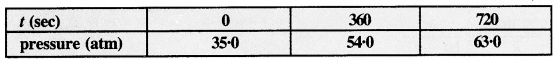Calculate the rate constant.
The decomposition reaction is of gaseous nature and expression of the rate equation for the reaction is :Question 21.
The following data were obtained during first order thermal decomposition of SO2Cl2 at a constant volume.
SO2Cl2 (g) → SO2 (g) + Cl2(g)Calculate the rate of the reaction when the total pressure is 0·65 atm. (C.B.S.E. Sample Paper 2011)Question 22.
The rate constant for the decomposition of N2O5 at various temperatures is given below :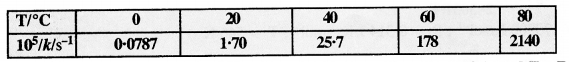Draw a graph between In k and 1/T and calculate the values of A and Ea. Predict the rate constant at 30° and 50°C.
To draw the plot of log K versus 1/T, we can re-write the given data as follows :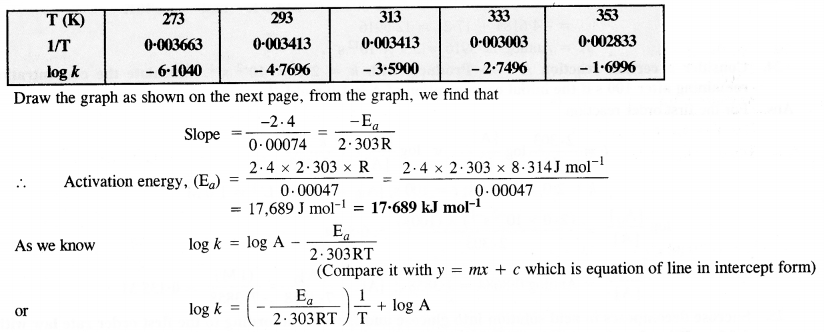Question 23.
The rate constant for the decomposition of a hydrocarbon is 2.418 × 10-5 s-1 at 546 K. If the energy of activation is 179.9 kJ/mol, what will be the value of pre-exponential factor.

Question 24.
Consider a certain reaction A → Products with k = 2·0 x 10-2 s-1. Calculate the concentration of A remaining after 100 s if the initial concentration of A is 1·0 mol L-1.
For the first order reaction :

Question 25.
Sucrose decomposes in acid solution into glucose and fructose according to the first order rate law with t1/2 = 3·0 hrs. What fraction of the sample of sucrose remains after 8 hours ? (C.B.S.E. Sample Paper 2011)Question 26.
The decomposition of a hydrocarbon follows the equation :
k = (4·5 x 1011 s-1)e-28000k/T.
Calculate the energy of activation (Ea).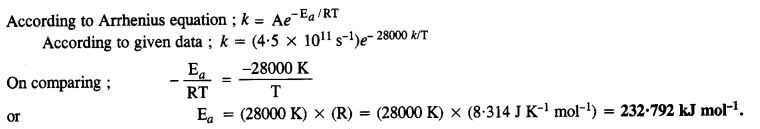Question 27.
The rate constant for the first order decomposition of H2O2 is given by the following equation :
log k = 14·34 – 1·25 x 104K/T.
Calculate the Ea for the reaction. At what temperature will the half life period be 256 minutes ?Question 28.
The decomposition of A into product has value of k as 4·5 x 103 s-1 at 10°C and energy of activation 60 kJ mol-1. At what temperature would k be 1·5 x 104 s-1 ? (C.B.S.E. Sample Paper 2011)
According to Arrhenius equation,Question 29.
The time required for 10% completion of a first order reaction at 298 K is equal to that required for its 25% completion at 308 K. If the value of A is 4 x 1010s-1, calculate k at 318 K and Ea.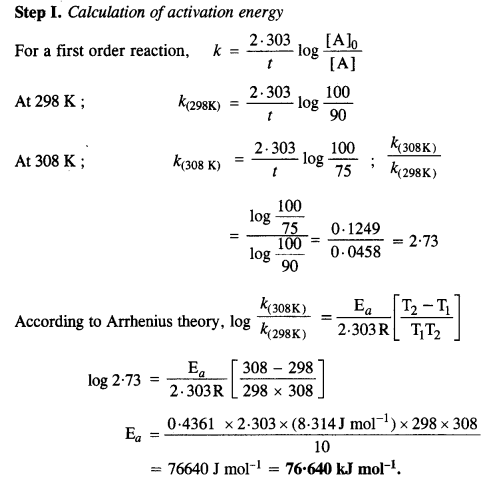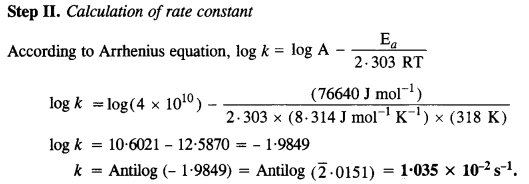Question 30.
The rate of a particular reaction quadruples when the temperature changes from 293 K to 313 K. Calculate the energy of activation of the reaction (R = 8·314 JK-1 mol-1). (C.B.S.E. Outside Delhi 2013)
According to Arrhenius equation,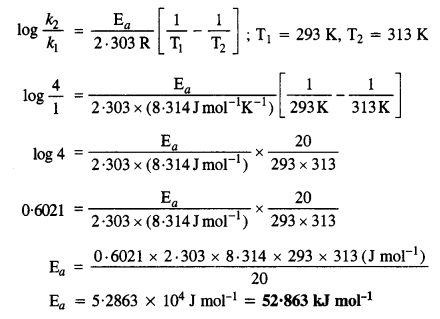All Chapter NCERT Solutions For Class 12 Chemistry

—————————————————————————–

All Subject NCERT Solutions For Class 12

*************************************************

I think you got complete solutions for this chapter. If You have any queries regarding this chapter, please comment on the below section our subject teacher will answer you. We tried our best to give complete solutions so you got good marks in your exam.

If these solutions have helped you, you can also share ncertsolutionsfor.com to your friends.